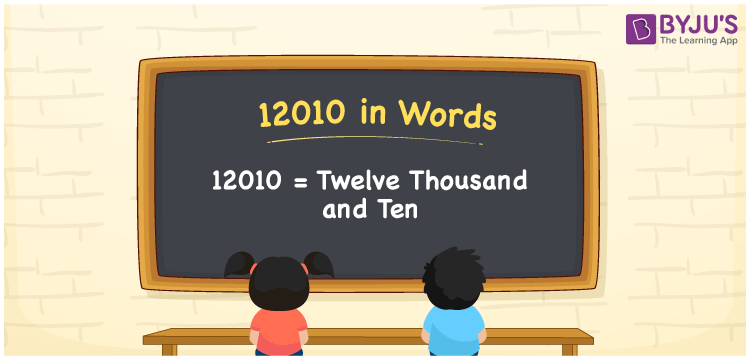# 12010 in Words

The number name of 12010 is Twelve Thousand and Ten. For instance, Priya purchased a dressing table worth Rs. 12010, then we can say, “Priya purchased a dressing table worth Rupees Twelve Thousand and Ten”. Place value chart is essential while converting the numbers into words. Hence, using a place value chart is beneficial to write the numerical name of any number. The number 12010 is a cardinal number. Let us glance at how to write the number name for 12010 in English words here.

 12010 in Words Twelve Thousand and Ten Twelve Thousand and Ten in numerical form 12010

## 12010 in English Words

Generally, we write the numbers in words using the letters of the English alphabet. Hence, the number 12010 in words is written as Twelve Thousand and Ten.## How to Write 12010 in Words?

Using a place value chart, we can convert the number 12010 to words with ease. We must create a table of 5 columns since 12010 is a five-digit number. The place value chart for the number 12010 along with the expanded form is given below.

 Ten Thousands Thousands Hundreds Tens Ones 1 2 0 1 0

Therefore, we can write the expanded form as:

1 x 10000 + 2 x 1000 + 0 x 100 + 1 x 10 + 0 x 1

= 10000 + 2000 + 0 + 10 + 0

= 10000 + 2000 + 10

= 12010

= Twelve Thousand and Ten

Hence, 12010 in words is written as Twelve Thousand and Ten

Interesting way of writing 12010 in words

1 = One

12 = Twelve

120 = One Hundred and Twenty

1201 = One Thousand Two Hundred One

12010 = Twelve Thousand and Ten

Thus, the word form of the number 12010 is Twelve Thousand and Ten

12010 is a natural number that precedes 12011 and succeeds 12009

• 12010 in words – Twelve Thousand and Ten
• Is 12010 an odd number? – No
• Is 12010 an even number? – Yes
• Is 12010 a perfect square number? – No
• Is 12010 a perfect cube number? – No
• Is 12010 a prime number? – No
• Is 12010 a composite number? – Yes

## Frequently Asked Questions on 12010 in Words

Q1

### How to write 12010 in words?

12010 in words is Twelve Thousand and Ten.
Q2

### Simplify 11000 + 1010, and express in words.

Simplifying 11000 + 1010, we get 12010. Thus, 12010 in words is Twelve Thousand and Ten.
Q3

### Write Twelve Thousand and Ten in numbers.

Twelve Thousand and Ten in numbers is 12010.